You've successfully subscribed to Better Data Science
Great! Next, complete checkout for full access to Better Data Science
Welcome back! You've successfully signed in
Success! Your account is fully activated, you now have access to all content.

# 3 Ways to Tune Hyperparameters of Machine Learning Models with Python#### From scratch to Grid Search — hands-on examples included.

Machine learning models can be quite accurate out of the box. But more often than not, the accuracy can improve with hyperparameter tuning.

Hyperparameter tuning is a lengthy process of increasing the model accuracy by tweaking the hyperparameters — values that can’t be learned and need to be specified before the training.

Today you’ll learn three ways of approaching hyperparameter tuning. You’ll go from the most manual approach towards a `GridSearchCV` class implemented with the Scikit-Learn library.

There’s no need to go crazy here. A simple dataset will do. You’ll work with the Iris dataset loaded straight from the web.

Library-wise, you’ll need Pandas to work with data, and a couple of classes/functions from Scikit-Learn. Here’s how to load in the libraries and the dataset:

``````import pandas as pd
from sklearn.tree import DecisionTreeClassifier
from sklearn.model_selection import train_test_split, GridSearchCV
from sklearn.metrics import accuracy_score, confusion_matrix

Calling the `head()` function will show the following data frame subset: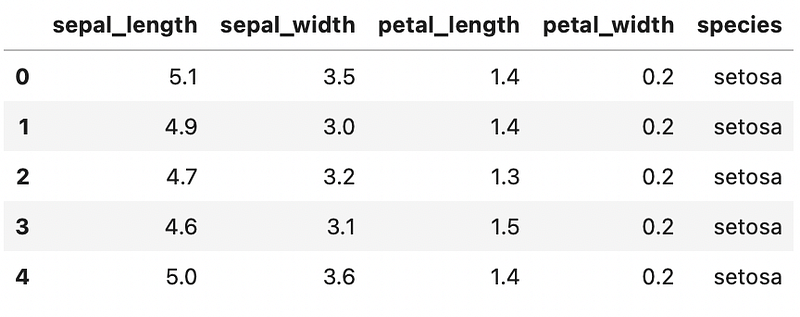Image 1 — Head of Iris dataset (image by author)

The dataset is as clean as they come, so there’s no need for additional preparation. Next, you’ll split it into training and testing subsets. Here’s how:

``````X = iris.drop('species', axis=1)
y = iris['species']

X_train, X_test, y_train, y_test = train_test_split(X, y, test_size=0.25)``````

Finally — let’s build a default model. It’ll show you how accurate the model with the default hyperparameters is, and it will serve as a baseline which the tweaked models should outperform.

Here’s how to train a Decision Tree model on the training set, obtain accuracy score and confusion matrix:

``````model = DecisionTreeClassifier()
model.fit(X_train, y_train)
preds = model.predict(X_test)

print(f'Accuracy = {round(accuracy_score(y_test, preds), 2)}')
print()
print(confusion_matrix(y_test, preds))``````

The corresponding accuracy and confusion matrix are shown below: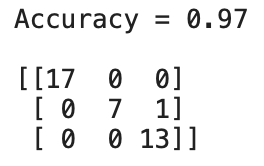Image 2 — Baseline model accuracy and confusion matrix (image by author)

In a nutshell — you want a model with more than 97% accuracy on the test set. Let’s see if hyperparameter tuning can do that.

## Manual hyperparameter tuning

You don’t need a dedicated library for hyperparameter tuning. But it’ll be a tedious process.

Before starting, you’ll need to know which hyperparameters you can tune. You can find the entire list in the library documentation. Here is the documentation page for decision trees. You’ll optimize only for the three in this article. These are:

• `criterion` – function which measures the quality of the split, can be either gini (default) or entropy
• `splitter` – a strategy for choosing a split at each node, can be either best (default) or random
• `max_depth` – a maximum depth of a tree, an integer value

You can define a set of hyperparameter values as a dictionary (key-value pairs) and then build separate models from them. Here’s how:

``````# 3 sets of hyperparameters
params_1 = {'criterion': 'gini', 'splitter': 'best', 'max_depth': 10}
params_2 = {'criterion': 'entropy', 'splitter': 'random', 'max_depth': 1000}
params_3 = {'criterion': 'gini', 'splitter': 'random', 'max_depth': 100}

# 3 separate models
model_1 = DecisionTreeClassifier(**params_1)
model_2 = DecisionTreeClassifier(**params_2)
model_3 = DecisionTreeClassifier(**params_3)

model_1.fit(X_train, y_train)
model_2.fit(X_train, y_train)
model_3.fit(X_train, y_train)

# 3 separate prediction sets
preds_1 = model_1.predict(X_test)
preds_2 = model_3.predict(X_test)
preds_3 = model_2.predict(X_test)

print(f'Accuracy on Model 1 = {round(accuracy_score(y_test, preds_1), 5)}')
print(f'Accuracy on Model 2 = {round(accuracy_score(y_test, preds_2), 5)}')
print(f'Accuracy on Model 3 = {round(accuracy_score(y_test, preds_3), 5)}')``````

Here are the corresponding accuracies: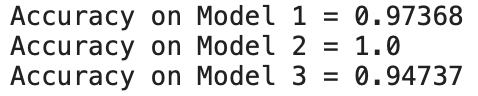Image 3 — Accuracies of manually tuned models (image by author)

To conclude — you’ve already managed to outperform the baseline model, but this approach isn’t scalable. Imagine if you wanted to test for 1000 combinations, which is actually a small number — writing code in this way isn’t a way to go. Let’s improve it next.

## Loop-based hyperparameter tuning

You can improve the previous solution by specifying possible hyperparameter values inside a list. There’ll be as many lists as there are hyperparameters. The model is then trained and evaluated inside a nested loop.

Here’s an example code snippet:

``````# Define parameter possibilities as lists
p_criterion = ['gini', 'entropy']
p_splitter = ['best', 'random']
p_max_depth = [1, 10, 100, 1000]
# The scores will go here
results = []

# Nested loops - we need to test for all combinations
for criterion in p_criterion:
for splitter in p_splitter:
for max_depth in p_max_depth:
# Train the model
model = DecisionTreeClassifier(
criterion=criterion,
splitter=splitter,
max_depth=max_depth
)
model.fit(X_train, y_train)
preds = model.predict(X_test)
# Append current results
results.append({
'Accuracy': round(accuracy_score(y_test, preds), 5),
'P_Criterion': criterion,
'P_Splitter': splitter,
'P_MaxDepth': max_depth
})

# Convert to Pandas DataFrame and sort descendingly by accuracy
results = pd.DataFrame(results)
results = results.sort_values(by='Accuracy', ascending=False)
results``````

As you can see, model accuracy on the test set and the respective hyperparameter values were stored as a dictionary in a list, which was later converted into a data frame. It’s easy to sort the data frame and see which hyperparameter combination did the best: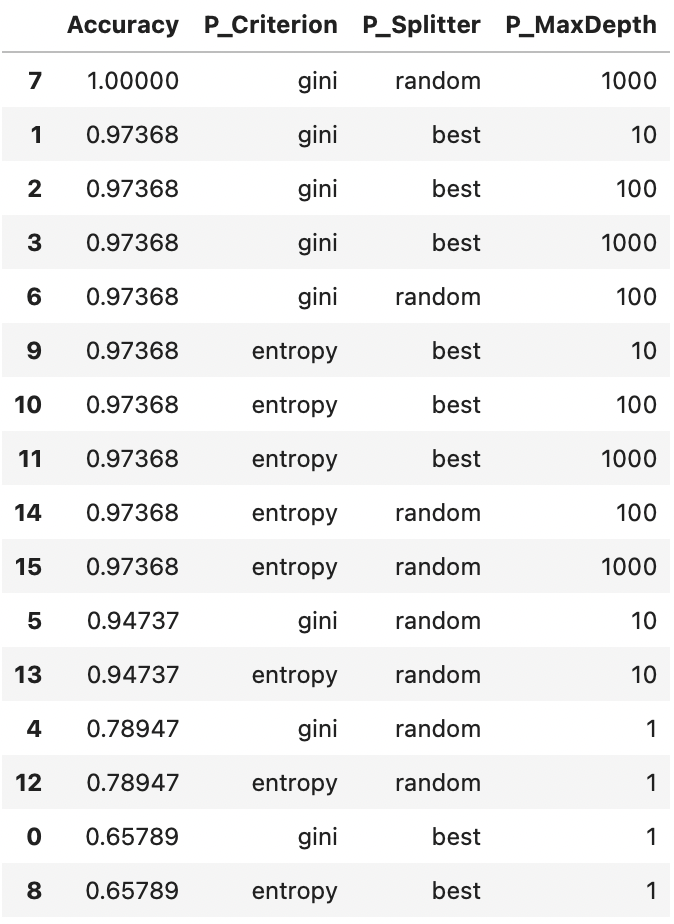Image 4 — Dataframe of scores and hyperparameters for manually tuned models(image by author)

To conclude — this approach works great, but you’re doomed to use nested loops. It’s okay for three hyperparameters, but imagine optimizing for ten. There must be a better way.

## Hyperparameter tuning with GridSearch

The `GridSearchCV` class comes with Scikit-Learn, and it makes hyperparameter tuning a joy. It can take a long time to optimize (nothing to do with the class), but you’re free from writing things manually.

You’ll need to declare a hyperparameter space as a dictionary, where each key is the name of the hyperparameter, and its value is a list of possible values. You can then use the `GridSearchCV` class to find an optimal set by calling the `fit()` function.

There’s also a benefit of built-in cross-validation with this approach, eliminating the “chance” from the results.

Here’s the entire code snippet:

``````model = DecisionTreeClassifier()
params = {
'criterion': ['gini', 'entropy'],
'splitter': ['best', 'random'],
'max_depth': [1, 10, 100, 1000]
}

clf = GridSearchCV(
estimator=model,
param_grid=params,
cv=10,  # 10-fold cross validation
n_jobs=-1  # run in parallel
)
clf.fit(X_train, y_train)``````

You can then store the results in a Pandas data frame (for easier inspection) — here’s how:

``````cv_results = pd.DataFrame(clf.cv_results_)

And here’s how the part of this data frame looks like: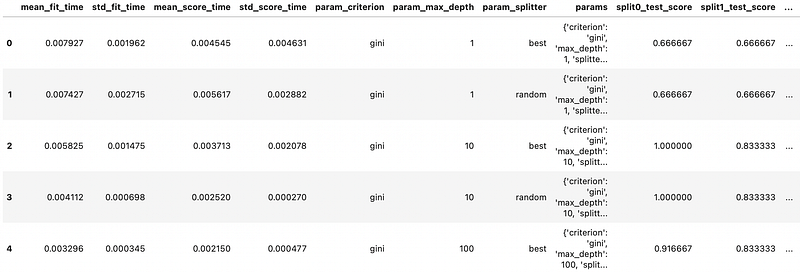Image 5 — Grid search parameter Dataframe (image by author)

Let’s filter this data frame to keep only the columns of interest — average test score and used hyperparameter values and sort by the average test score:

``````cv_results = cv_results[['mean_test_score', 'param_criterion', 'param_splitter', 'param_max_depth']]
cv_results.sort_values(by='mean_test_score', ascending=False)
``````

Here are the results: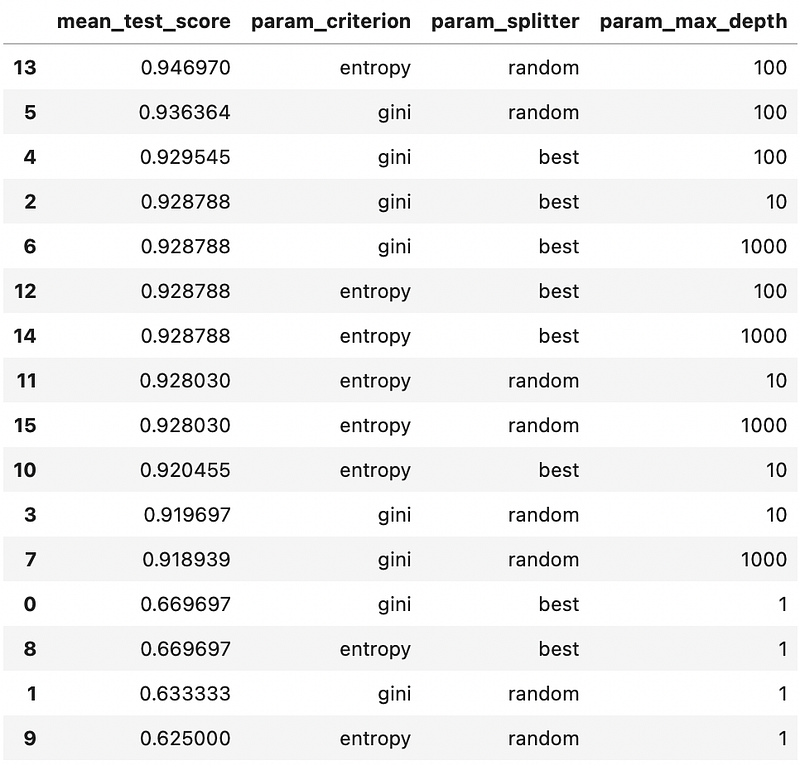Image 6 — Dataframe of scores and hyperparameters for model tuned with GridSearch (image by author)

That’s a good approach if you’re interested in examining multiple combinations. An easier way exists if you only want the best values:

``clf.best_params_``

This property returns a dictionary: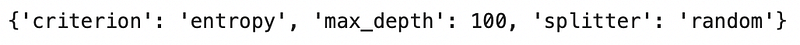Image 7 — Best hyperparameters (image by author)

You can pass the dictionary directly to the machine learning model (use unpacking — `**dict_name`).

And that’s how easy it is to find optimal hyperparameters for a machine learning algorithm. Let’s wrap things up next.

## Conclusion

The last approach will get the job done most of the time. You’re free to do the optimization manually, but what’s the point?

Grid search can take a lot of time to finish. Let’s say you have 5 parameters with 5 possible values. That’s 5ˆ5 of possible combinations (3125). Add cross-validation into the picture (let’s say 10-fold), and that is 31250 models you need to train and evaluate.

For these cases, a Randomized grid search might be a better option. Code-wise it works the same as the non-randomized one, so that’s why it wasn’t covered today.